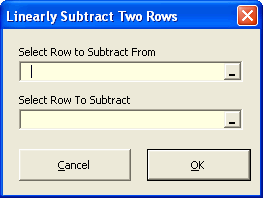### Strutt Help

Linear Subtraction    1/1, 1/3

Strutt|Lin Subtract Rows function prompts for two rows, one to subtract from, and the one to subtract with the result being placed in the active row.Strutt implements the subtraction as:

x = a-b

Strutt places a '+' in Column A of the basic noise level, a '-' in Column A of the row that is subracted from it, and an '=' in the results row.